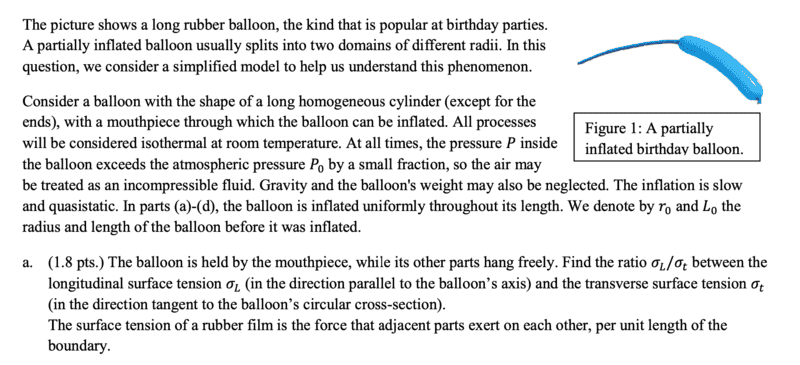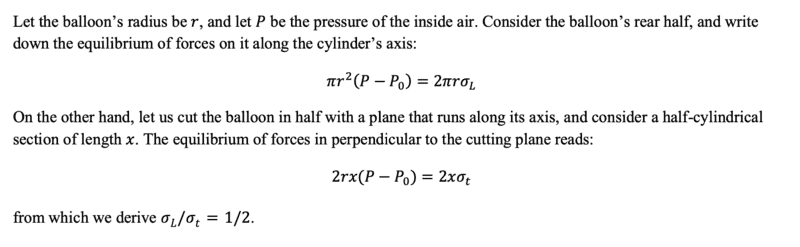# Problem on Pressure due to Surface tension

• phantomvommand
In summary, the method to solve this problem involves equating forces along different portions of the balloon, with one portion being a circular cross section and the other being a rectangular cross section. There is some ambiguity in the definition of ##\sigma_L## and ##\sigma_t##, but it ultimately does not affect the final result. The issue lies in the fact that this is not a traditional surface tension problem, but rather a problem involving internal stresses in the rubber of the balloon.

#### phantomvommand

Homework Statement
Relevant Equations
##\gamma = \frac F L##
##P = \frac F A##The method to solving this is to equate forces along a portion of the balloon through which ##\sigma_L## acts, and another portion through which ##\sigma_t## acts. The former potion should be a circular cross section of the cylinder, while the latter will be a rectangular cross section. You will thus get the following:I did exactly the above, except that instead of having ##2\pi r \sigma_L## and ##2x\sigma_t## on the RHS, I had ##4\pi r \sigma_L## and ##4x\sigma_t##. Am I right on this? Because I think that in either case, there are 2 surfaces (inner surface of balloon and outer surface of balloon), resulting in double the force exerted by surface tension.

In the problem they've defined ##\sigma_L## and ##\sigma_t## as forces per unit length of the boundary between two portions in the longitudinal and hoop directions respectively. With that definition there's no factor of 2. Although you're right to be a little skeptical, because there are indeed two surfaces and usually the longitudinal surface tension ##\gamma_L## for instance would be defined such that e.g. ##\pi r^2 P' = 4\pi r \gamma_L##, i.e. with the factor of two included.

The reason for the ambiguity is that it's not really a surface tension problem. It's really instead internal stresses in the rubber holding the thing together (although there is a strong analogy). For actual surface tension problems, ##\gamma## is defined thermodynamically as ##\gamma = \partial E / \partial A## where ##E## is the interface energy between two phases and ##A## is the total area of the interface between those two phases, and it's important to account for all of the interfaces.

Here it doesn't really matter how you define ##\sigma_L## and ##\sigma_t## just so long as you're consistent because at the end you're taking a ratio. So I wouldn't worry about it too much!•phantomvommand